Finding the First Login and Last Logout Times of Employees

In this article, you’ll learn how to find the first login and last logout times of employees by using Microsoft Excel functions. To solve this query, we use MAX function for both.

Let’s take an example,

We have data which contain IDS, Login and logout time details of various employees. Each employee can login and log out several times a day. We want to find the first time each employee logged in and the last time they logged out.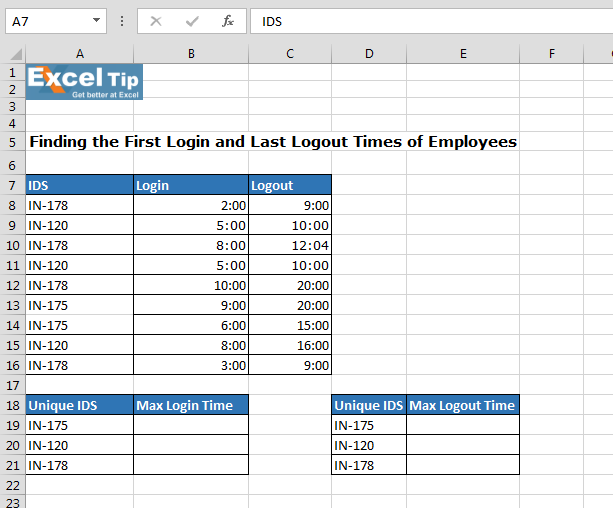• Enter the formula in cell B19
• =1/MAX((A19=\$A\$8:\$A\$16)*(\$B\$8:\$B\$16<>0)*(1/\$B\$8:\$B\$16))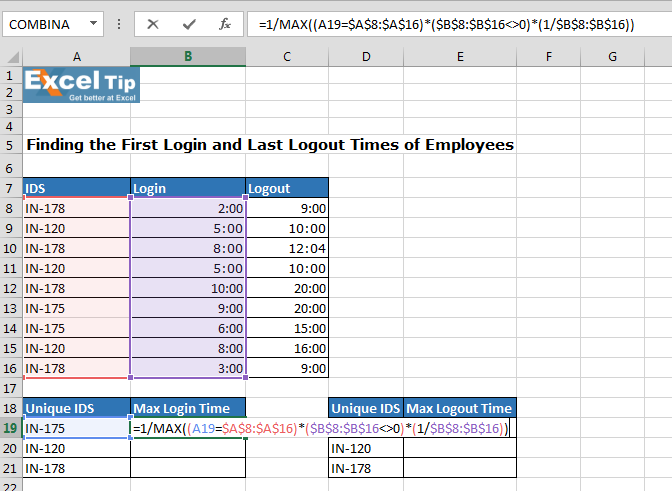Note: - This is an array formula.

• Press Ctrl+Shift+Enter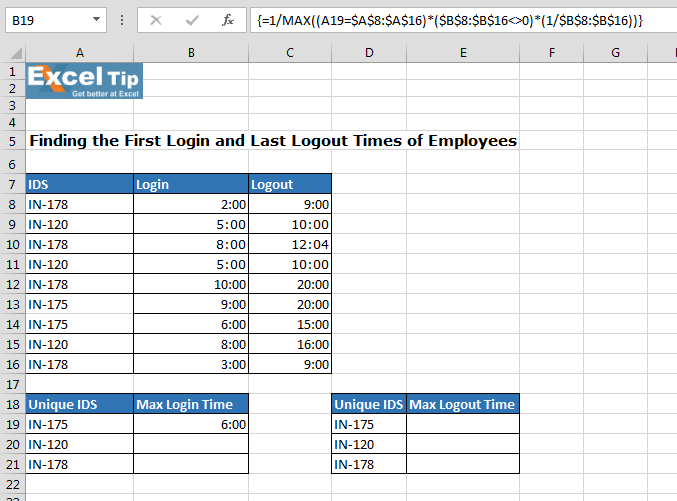• Copy the same formula in cell B20:B21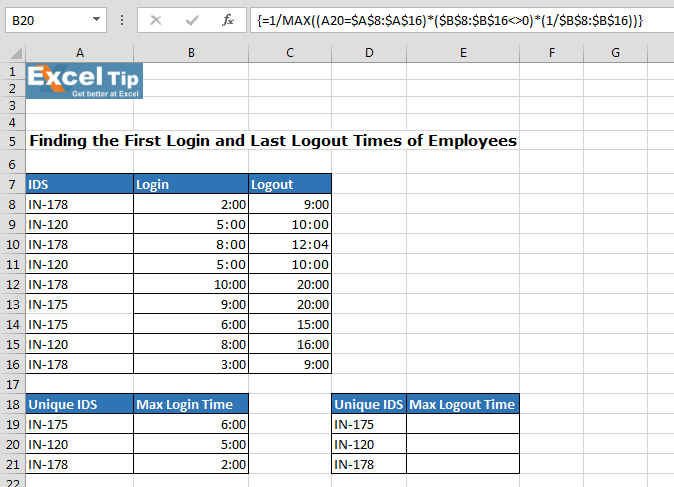Now, we will calculate Log out time. Follow below given steps:-

• Enter the formula in cell E19
• =MAX((\$A\$8:\$A\$16=D19)*(\$C\$8:\$C\$16))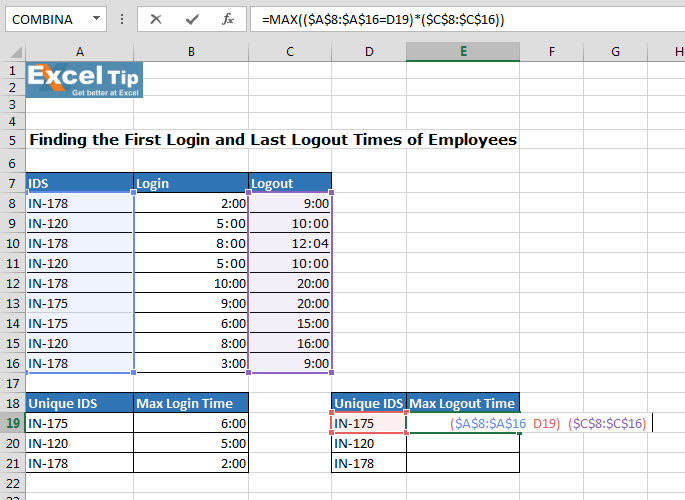• Press Ctrl+Shift+Enter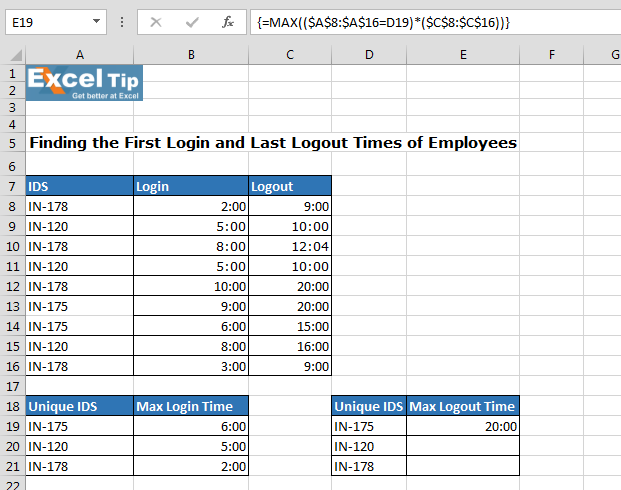• Copy the formula in cell E20:E21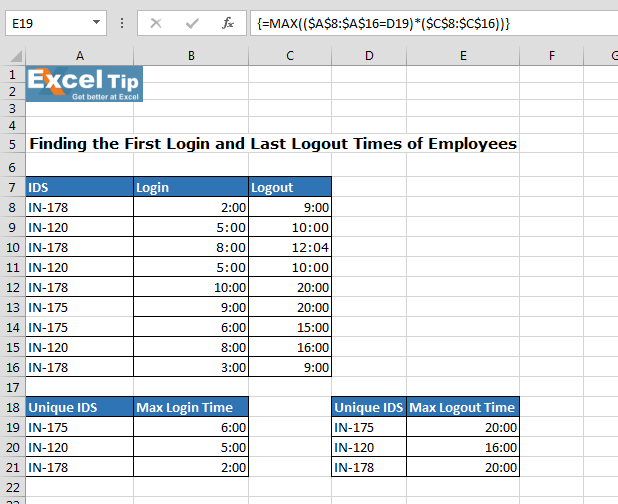You can see in the above image that we have used MAX function to get the minimum login time and, to get the maximum log out time, we have used MAX function.

In this way, we can find the first login and last logout times for each employee by using Microsoft Excel function.We would love to hear from you, do let us know how we can improve, complement or innovate our work and make it better for you. Write us at info@exceltip.com

1.